# Odd One Out Practice 2

In this practice exercise, students will identify an image that does not fit in with the rest of the group. The focus will be on direction, position and number, so students will need to look at where shapes are, which direction they are facing, and how many there are under timed conditions.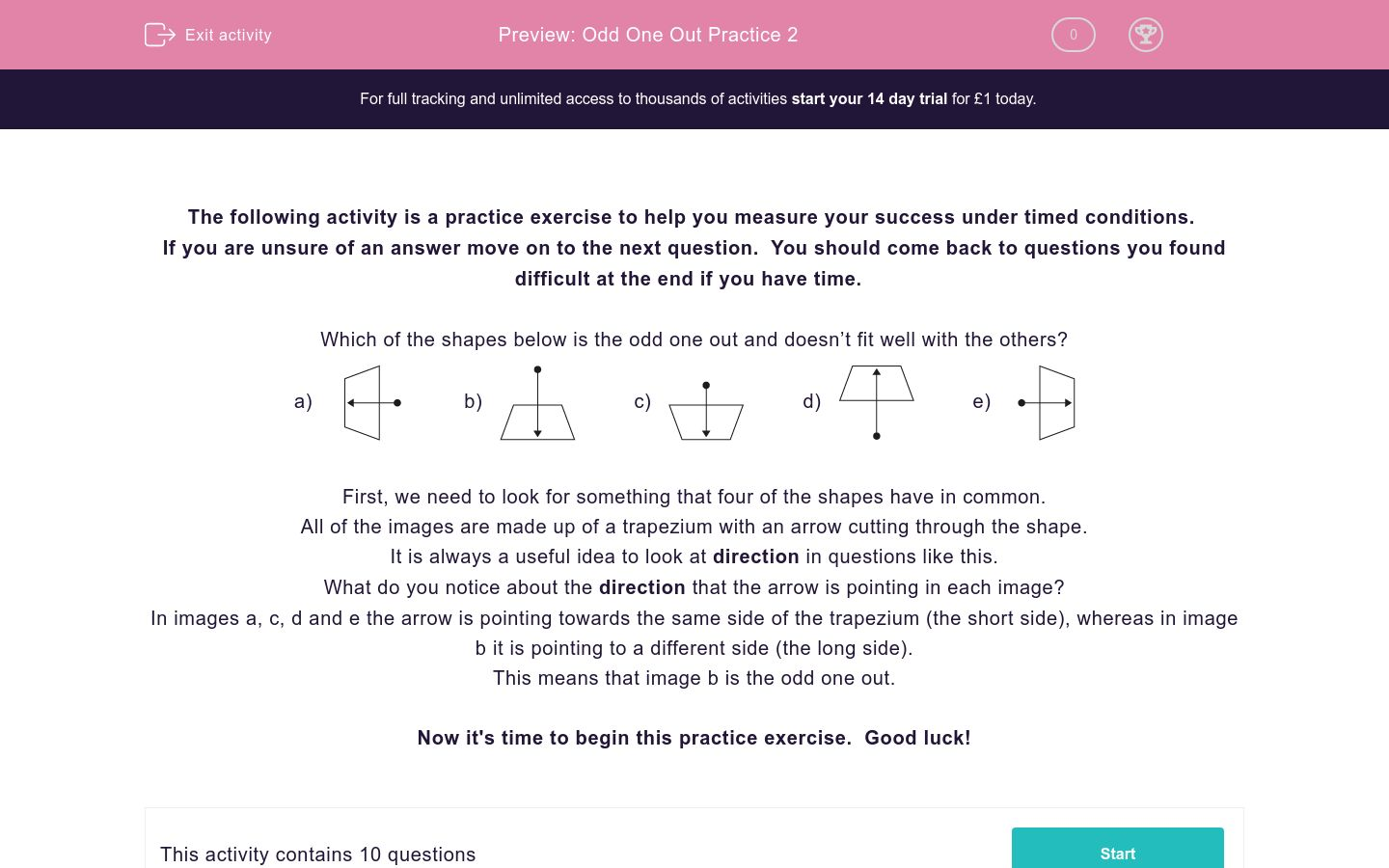Key stage:  KS 2

Curriculum topic:   Exam-Style Questions: Spatial & Non-Verbal Reasoning

Curriculum subtopic:   Exam-Style Questions: Odd One Out

Difficulty level:### QUESTION 1 of 10

If you are unsure of an answer move on to the next question.  You should come back to questions you found difficult at the end if you have time.

Which of the shapes below is the odd one out and doesn’t fit well with the others?

a)b)c)d)e)First, we need to look for something that four of the shapes have in common.

All of the images are made up of a trapezium with an arrow cutting through the shape.

It is always a useful idea to look at direction in questions like this.

What do you notice about the direction that the arrow is pointing in each image?

In images a, c, d and e the arrow is pointing towards the same side of the trapezium (the short side), whereas in image b it is pointing to a different side (the long side).

This means that image b is the odd one out.

Now it's time to begin this practice exercise.  Good luck!

Which of the images below is the odd one out?

a)b)c)d)e)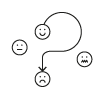Which of the images below is the odd one out?

a)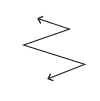b)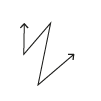c)d)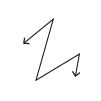e)Which of the images below is the odd one out?

a)b)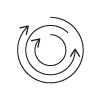c)d)e)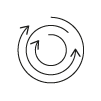Which of the images below is the odd one out?

a)b)c)d)e)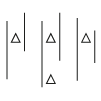Which of the images below is the odd one out?

a)b)c)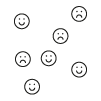d)e)Which of the images below is the odd one out?

a)b)c)d)e)Which of the images below is the odd one out?

a)b)c)d)e)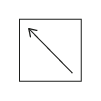Which of the images below is the odd one out?

a)b)c)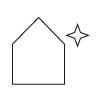d)e)Which of the images below is the odd one out?

a)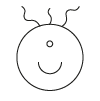b)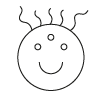c)d)e)Which of the images below is the odd one out?

a)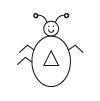b)c)d)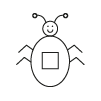e)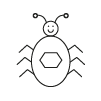• Question 1

Which of the images below is the odd one out?

d)EDDIE SAYS
There are a lot of faces showing different emotions here- I hope you didn\'t get emotional about it! As is often the case, the arrows here can POINT us to the correct answer. We need to look at where the arrows are coming from and going to. In options a, b, c and e the arrow is going from a happy face to a sad face. In option d the arrow is working in reverse, going from a sad face to a happy face. This makes option d the odd one out.
• Question 2

Which of the images below is the odd one out?

c)EDDIE SAYS
As a detective, there is sometimes more than one route to solve a problem. This is the case in this question, although they both involve counting. We could work out the odd one out by either counting the number of lines making up each arrow or by counting the number of corners in each arrow - both of these options will get us to the correct answer. Options a, b, d and e all have arrows made of 4 lines and 3 corners, whereas option c has an arrow made of 7 lines and 6 corners. This makes option c the odd one out.
• Question 3

Which of the images below is the odd one out?

d)EDDIE SAYS
We have to look carefully at the directions in which the arrows are pointing in this question. In options a, b, c and e there is a clockwise arrow in the middle, followed by an anti-clockwise arrow and then another clockwise arrow on the outside. Phew, there are lots of directions and lines to consider here! In option d there is an anti-clockwise arrow in the middle, then a clockwise, ending with another anti-clockwise. As the pattern is reversed here, this makes option d the odd one out.
• Question 4

Which of the images below is the odd one out?

d)EDDIE SAYS
There is a relationship in action here between the number of triangles and the number of lines. In options a, b, c and e there are two less triangles than the number of lines. For example, option a has 5 lines and 3 triangles and option b has 3 lines and 1 triangle. This is not the case in option d, which has an equal number of lines and triangles (2), making it the odd one out.
• Question 5

Which of the images below is the odd one out?

d)EDDIE SAYS
Here are some more faces showing a lot of emotions! There is a connection between the number of happy faces and the number of sad faces. In options a, b, c and e there is one less sad face than happy face. In option d there are an equal amount of sad and happy faces which does not fit with the rule. This makes option d the odd one out.
• Question 6

Which of the images below is the odd one out?

d)EDDIE SAYS
There are lots of things you could consider here, but you need to find the one that is the top clue and will enable you to reach the correct answer. There is a relationship between the number of sides of the main shape and the numbers of T\'s inside each. Option a has 7 sides and 4 T\'s inside. Option b has 5 sides and 2 T\'s inside. These both have 3 less T\'s than they do sides. You can test this relationship with the other options, and it works for c and e. It does not work for option d, which has 6 sides and 4 T\'s inside. This isn\'t three less T\'s than sides but 2, so this makes option d the odd one out.
• Question 7

Which of the images below is the odd one out?

a)EDDIE SAYS
It\'s time to switch into direction detective mode for this question! Each option has a square with an arrow inside. Where are the arrows pointing to? In options b, c, d and e the arrows are pointing to a corner of the square, but in option a they are pointing to a side. This makes option a the odd one out. Don\'t get SQUARE eyes detective - just 3 questions to go!
• Question 8

Which of the images below is the odd one out?

c)EDDIE SAYS
Here you need to spot the relationship between the number of sides of the big shapes and the number of points on the star outside each. Can you work it out? Option a has a 3-sided shape with a 3-pointed star on the outside. Option b has a 4-sided shape with a 4-pointed star outside. The number of sides of the main shape appears to match the number of points on the star. Can you test this with the other options? If you do, you will find that options d and e also follow this pattern but option c does not. Option c has a 5-sided shape with a 4-pointed star which doesn\'t match and makes it the odd one out.
• Question 9

Which of the images below is the odd one out?

d)EDDIE SAYS
There are a few bad hair days going on here for these aliens! Can you spot the connection between the number of strands of hair and the number of eyes? In options a, b, c and e there are 2 more strands of hair than the number of eyes. For example, the alien in option a has 1 eye and 3 strands of hair. This connection is not maintained in option d, which has 3 strands of hair and 2 eyes, which makes it the odd alien out!
• Question 10

Which of the images below is the odd one out?

c)EDDIE SAYS
Try not to get itchy looking at these bugs! There is a connection here between the number of sides of the shapes on the bugs\' bellies and the number of legs that each has. Did you spot it with your detective\'s microscope? Bug a has a 3-sided shape on its belly and 3 legs, whilst bug b has a 5-sided shape and 5 legs. It seems that the number of sides of the shape on their belly and the number of legs match. Can you check this out for the other options? If you do, you will find that this relationship fits for option d and e but not for option c. Bug c has a 4-sided shape on its belly but has 5 legs, so it is the odd bug out! Great work top detective! Remember to always check for position, direction and something to count in questions of this type as these will give you the best clues.
---- OR ----

Sign up for a £1 trial so you can track and measure your child's progress on this activity.

### What is EdPlace?

We're your National Curriculum aligned online education content provider helping each child succeed in English, maths and science from year 1 to GCSE. With an EdPlace account you’ll be able to track and measure progress, helping each child achieve their best. We build confidence and attainment by personalising each child’s learning at a level that suits them.

Get started